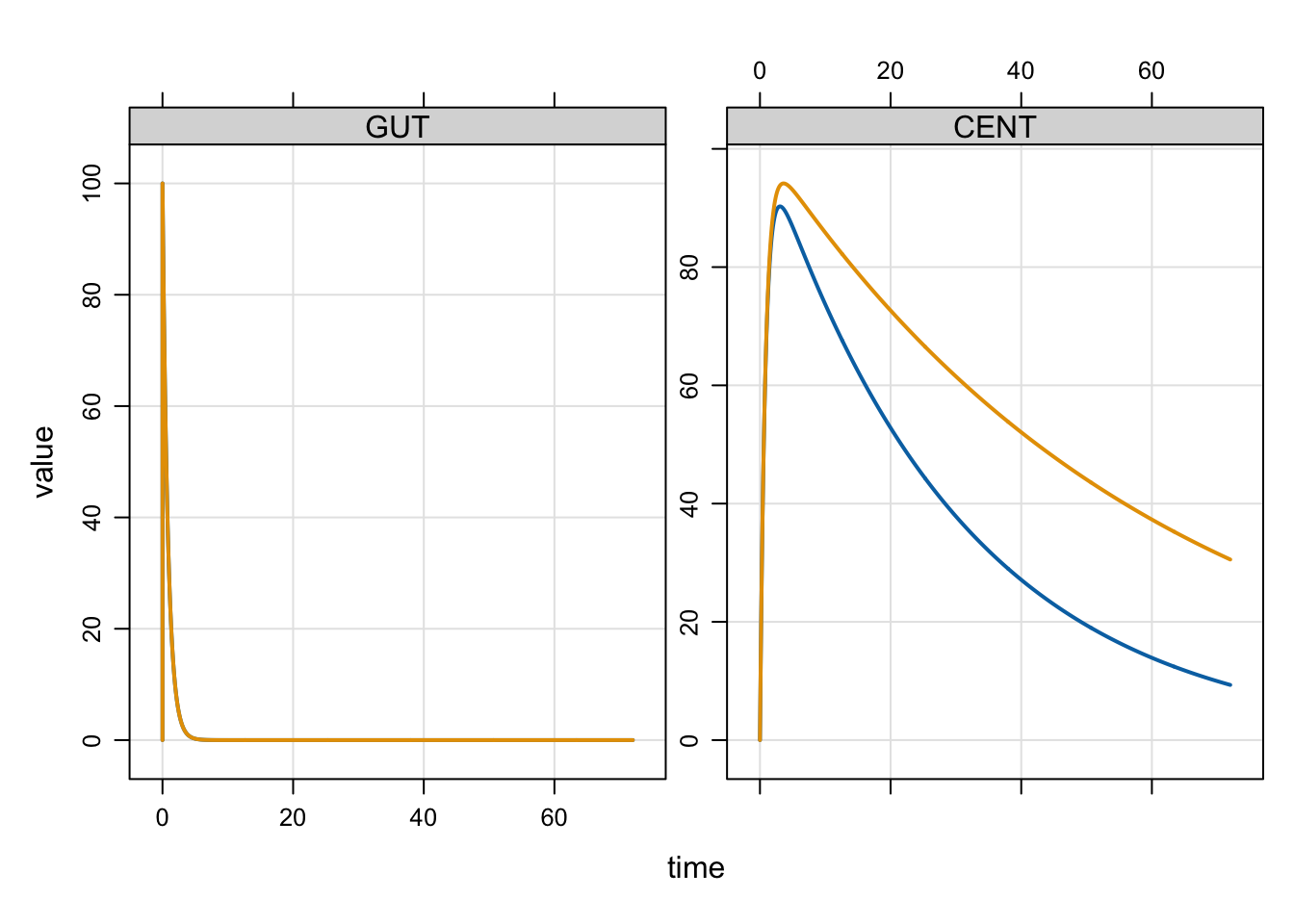# Mixture model example

In this post, we’ll look at how you can code up a mixture model in mrgsolve.

mixture model
Author

Kyle Baron

Published

January 1, 2017

In NONMEM, `\$MIXTURE` will allow you to estimate mixture models, where individuals are classified in to two or more populations with a certain probability. It is straightforward to simulate from models like these in your `mrgsolve` model code.

# 1 Two Populations

Let’s imagine there were two populations in the mixture model, with the second having smaller clearance than the first. In this example, we will develop some code for a simple model and then extend it to implement the mixture model component.

A simple model might be:

``````library(mrgsolve)
library(dplyr)

code <- '
\$PARAM TVCL = 1, V = 30, KA=1.2, POP = 1, THETA1 = 0.5

\$PKMODEL cmt="GUT CENT", depot=TRUE

\$MAIN
double CL = TVCL;

if(POP==2) CL = TVCL * THETA1;
'``````

In this model, we created a parameter for the population indicator (`POP`) and if `POP` is 2 then clearance is lower than it would be otherwise.

Compile this model and run

``mod <- mcode_cache("A", code) %>% update(end=72, delta=0.1)``
``Building A ... done.``
``idata <- data_frame(POP=c(1,2))``
``````Warning: `data_frame()` was deprecated in tibble 1.1.0.
``````e <- ev(amt=100)

mod %>% mrgsim(idata=idata,events=e) %>% plot``````The profile in pink was for `POP==2` or the lower clearance profile and blue was for `POP==1`.

## 1.1 Modify the model to simulate a population mixture

In the get-started example model, we hard-coded `POP` as a parameter and we had to supply the value of `POP` in the input data set (in this case, it was via `idata`).

For the mixture model, we want `POP` to be simulated and we want the simulated value to be 1 with a probability of, say, 0.8 and 2 with a probability of 0.2.

To make this happen, we need to simulate a binary variate for each individual. Random numbers are easy to simulate with `mrgsolve` when you use `\$PLUGIN`.

``````code <- '
\$PLUGIN Rcpp

\$MAIN
if(NEWIND <=1) {
int POP = 1 + R::rbinom(1,0.2);
}

\$CAPTURE POP
'

mod <- mcode_cache("B", code)``````
``Building B ... done.``

Here, we invoked the `Rcpp` plugin that allows us to call `R::binom(1,0.8)`. `R::binom` is just like the regular `R` version, but it only draws one variate (`n=1`).

Let’s test it out

``````set.seed(222)
out <- mrgsim(mod, nid=10000, end=-1)

``````  ID time POP
1  1    0   2
2  2    0   1
3  3    0   1
4  4    0   1
5  5    0   2
6  6    0   2``````

Here, we’ve got 20% of the people in the population with `POP` of 2:

``mean(out\$POP==2)``
`` 0.1973``

Now, let’s modify the model again to incorporate our random `POP` calculation with the PK model. I have also included a home-brewed `ETA` using `R::rnorm` as another example and to make the summary a little more interesting.

``````code <- '
\$PLUGIN Rcpp

\$PARAM TVCL = 1, V = 30, KA=1.2, THETA1 = 0.5

\$PKMODEL cmt="GUT CENT", depot=TRUE

\$MAIN
if(NEWIND <=1) {
int POP = 1 + R::rbinom(1,0.2);
double myETA = R::rnorm(0,sqrt(0.09));
}

double CL = TVCL;

if(POP==2) CL = TVCL * THETA1;

double CLi = CL*exp(myETA);

\$CAPTURE POP CL CLi
'

mod <- mcode_cache("C", code)``````
``Building C ... done.``

And simulate again

``````set.seed(444)
out <- mrgsim(mod,nid=10000, end=72, events=e,obsonly=TRUE)``````
``head(out)``
``````  ID time        GUT     CENT POP CL       CLi
1  1    0  0.0000000  0.00000   1  1 0.7642753
2  1    1 30.1194212 68.50511   1  1 0.7642753
3  1    2  9.0717953 86.89259   1  1 0.7642753
4  1    3  2.7323722 90.25855   1  1 0.7642753
5  1    4  0.8229747 89.17134   1  1 0.7642753
6  1    5  0.2478752 86.81173   1  1 0.7642753``````
``mean(out\$CL==0.5)``
`` 0.1977``
``````out %>%
filter(time==0) %>%
group_by(POP) %>%
summarise(N=n(), Median = median(CLi))``````
``````# A tibble: 2 × 3
POP     N Median
<dbl> <int>  <dbl>
1     1  8023  1.00
2     2  1977  0.499``````

# 2 Three (or more) Populations

There are probably several ways to simulate three populations. Here is one way. We’ll drop the PK model for now and focus on generating `POP`.

``````code <- '
\$PARAM p1 = 0.33, p2 = 0.6

\$PLUGIN Rcpp

\$MAIN
if(NEWIND <=1) {
double mixv = R::runif(0,1);
int POP = 1;
if(mixv > p1) POP = 2;
if(mixv > (p1+p2)) POP = 3;
}

\$CAPTURE POP mixv
'``````

Here’s what we did

• Code mixture probabilities in `\$PARAM`
• Draw a variate (`mixv`) from `uniform(0,1)`
• Determine `POP` based on the probabilities and `mixv`
• Remember: you must use `\$PLUGIN` for this to work

Now, let’s compile and test it out

``mod <- mcode_cache("D", code)``
``Building D ... done.``
``````set.seed(333)
out <- mrgsim(mod, nid=10000, end=-1)``````
``head(out)``
``````  ID time POP       mixv
1  1    0   2 0.46700066
2  2    0   1 0.08459815
3  3    0   3 0.97348527
4  4    0   2 0.57130558
5  5    0   1 0.02011937
6  6    0   2 0.72355739``````

And check that the population is properly configured

``out %>% as_tibble() %>% count(POP) %>% mutate(p = n/nrow(out))``
``````# A tibble: 3 × 3
POP     n      p
<dbl> <int>  <dbl>
1     1  3225 0.322
2     2  6053 0.605
3     3   722 0.0722``````

And we get back the 33% in population 1, 60% in population 2, and the remaining 7% in population 3.

As a final note: remember to call `set.seed()` prior to simulating anything random with `mrgsovle` in order for the results to be reproducible.